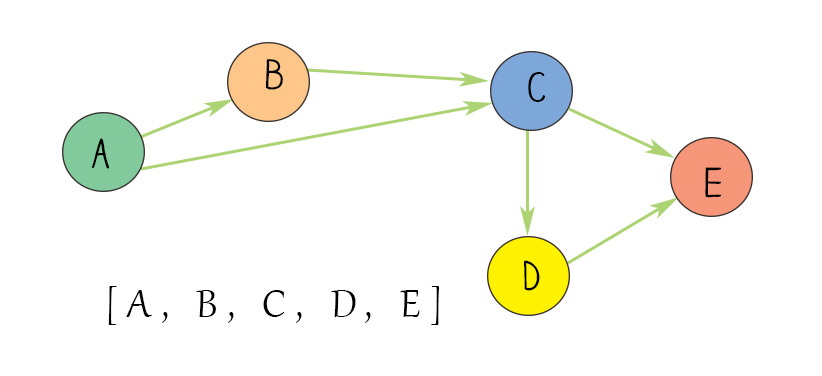Topological sort is one of graph problems. It is to find the order of vertices in a directed graph based on the edge directions. For example, an edge [uv] starts from u and ends at v. Another edge [mn] starts from m and ends at n. After applying topological sort, the sequence of vertices (u, v, m, n ) should satisfy all edge directions. In the following diagram, there are 5 edges. The sequence of (A, B, C, D, E) satisfies all directions of edges.Topological sort can be only applied to a directed graph that doesn’t have a cycle, called Directed Acyclic Graph(DAG). There are a few algorithms to implement topological sort. The commonly used ones are Depth first search and Kahn’s algorithm. The results are not necessary unique. But they all should meet the criteria to satisfy the edge directions.

Since it is a graph problem, you can make use of the exiting Graph class. Use addEdge method in Graph class so that you can create a graph quickly. In both DFS and Kahn’s algorithm, use a graph object as the input. The output is the list of vertices that is a topological sort list.

### Topological Sort DFS

Depth first search is the same as depth first search in a graph traversal. Starting from the source node, you call recursive method to visit its neighbor’s neighbor until call back.  It use a variable visited to track whether the node has been visited so that each node is visited once.

Another variable stack is used to store the result. After a recursive function returns, that means the node has reaches to the state that there is no unvisited target nodes in its neighbors. Push this node in the stack. At the end, reverse the stack, you will get the result.

## Python

### Kahn’s algorithm (BFS)

Kahn’s algorithm uses indegree and queue data structure. indegree is a hash map of all nodes and their counts. If a node is in another node’s neighbors, its indegree count increases by 1. If a node’s indegree count is 0, this node has no target nodes in its neighbors. It is the start node. Put this node in the queue.

Next use BFS by taking out the first item in the queue. Put it in the result list. Then use for loop to check its target nodes and reduce the target node’s indegree count by 1. When the node’s indegree count is 0, you can add the node to the queue. At the end, when the result list has all the nodes in a graph, you can return the result.